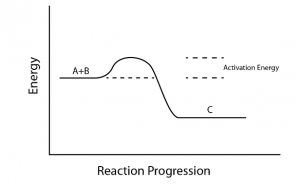# Activation Energy IIT JEE Study Material

Activation energy is one of the most important concepts to deal with in physical chemistry for JEE. It is a very important concept related to chemical kinetics. Some FAQs related to activation energy are underlined below Activation energy is the minimum energy required for the reacting species to form an intermediate (better known as activated complex). In other words, it is the potential energy barrier for the reacting species to cross in order to form products.The Arrhenius Eqn gives the quantitative relationship between the activation energy (Ea) and the rate (k) at which a reaction proceeds. The activation energy (Ea) can be evaluated from the given relation:

$\mathbf{k\;=\;A\;e\;^{\frac{-E_{a}}{RT}}}$

Where,

k = Reaction rate coefficient

R = Universal gas constant,

A = Frequency factor for the reaction,

T = Absolute temperature (K)

## Negative activation energy:

Sometimes with increasing temperature, there is a decrease in the rate of reaction. While following an exponential relationship so as the rate constant (k) can still be fit to an Arrhenius expression resulting in the negative values of activation energy (Ea).

Question: What are the different factors which affect the activation energy?